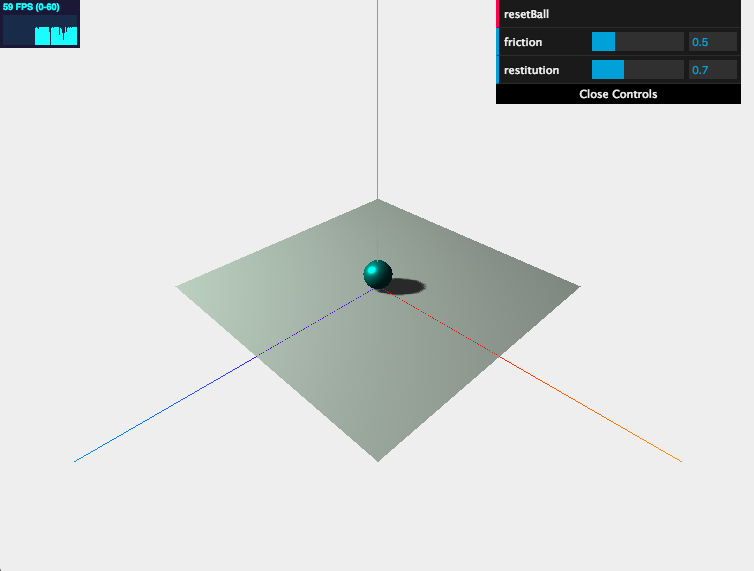#2019 iT 邦幫忙鐵人賽DAY 21
1
Modern Web

## 用 Three.js 來當個創世神 (20)：Cannon.js 基本練習Photo by Dawid Zawiła on Unsplash

## Hello Cannon.js## 程式碼解析

``````https://cdnjs.cloudflare.com/ajax/libs/cannon.js/0.6.2/cannon.min.js
``````

### 1. 建立物理世界

``````// 建立物理世界
world = new CANNON.World()

// 設定重力場為 y 軸 -9.8 m/s²
world.gravity.set(0, -9.8, 0)

// 碰撞偵測
``````

### 2. 建立動態球型剛體

``````// 建立球剛體
let sphereShape = new CANNON.Sphere(1)
let sphereCM = new CANNON.Material()
let sphereBody = new CANNON.Body({
mass: 5,
shape: sphereShape,
position: new CANNON.Vec3(0, 10, 0),
material: sphereCM
})
``````

### 3. 建立靜態地板剛體

``````// 建立地板剛體
let groundShape = new CANNON.Plane()
let groundCM = new CANNON.Material()
let groundBody = new CANNON.Body({
mass: 0,
shape: groundShape,
material: groundCM
})
// setFromAxisAngle 旋轉 x 軸 -90 度
groundBody.quaternion.setFromAxisAngle(new CANNON.Vec3(1, 0, 0), -Math.PI / 2)
``````

### 4. 設定碰撞時交互作用屬性

``````let sphereGroundContact
sphereGroundContact = new CANNON.ContactMaterial(groundCM, sphereCM, {
friction: 0.5,
restitution: 0.7
})
``````

### 5. 建立球體與地板的網格

``````// 地板網格
let groundGeometry = new THREE.PlaneGeometry(20, 20, 32)
let groundMaterial = new THREE.MeshLambertMaterial({
color: 0xa5a5a5,
side: THREE.DoubleSide
})
let ground = new THREE.Mesh(groundGeometry, groundMaterial)
ground.rotation.x = -Math.PI / 2

// 球網格
let sphereGeometry = new THREE.SphereGeometry(1, 32, 32)
let sphereMaterial = new THREE.MeshStandardMaterial({ color: 0x33aaaa })
sphere = new THREE.Mesh(sphereGeometry, sphereMaterial)
``````

### 6. 更新物理世界動畫

``````const timeStep = 1.0 / 60.0 // seconds

function render() {
world.step(timeStep)
if (sphere) {
sphere.position.copy(sphereBody.position)
sphere.quaternion.copy(sphereBody.quaternion)
}

requestAnimationFrame(render)
renderer.render(scene, camera)
}
``````